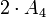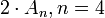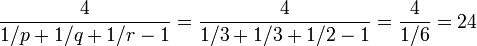# Difference between revisions of "Special linear group:SL(2,3)"

Jump to: navigation, search

This article is about a particular group, i.e., a group unique upto isomorphism. View specific information (such as linear representation theory, subgroup structure) about this group
View a complete list of particular groups (this is a very huge list!)[SHOW MORE]

## Definition

The special linear group$SL(2,3)$ is defined in the following equivalent ways:

• It is the special linear group of degree two over a field of three elements. In other words, it is the group of$2 \times 2$ matrices with determinant$1$ over the field of three elements.
• It is the double cover of alternating group$2 \cdot A_4$, i.e., it is the double cover of alternating group:A4.
• It is a binary von Dyck group with the parameters$(3,3,2)$, i.e., it has the presentation:$\langle a,b,c \mid a^3 = b^3 = c^2 = abc \rangle$.

It is a group of order$24$.

## Arithmetic functions

Function Value Similar groups Explanation
order (number of elements, equivalently, cardinality or size of underlying set) 24 groups with same order As$SL(2,q)$,$q = 3$:$q^3 - q = 3^3 - 3 = 24$
As$2 \cdot A_n, n = 4$:$n! = 4! = 24$
As binary von Dyck group with parameters$(p,q,r) = (3,3,2)$:$\frac{4}{1/p + 1/q + 1/r - 1} = \frac{4}{1/3 + 1/3 + 1/2 - 1} = \frac{4}{1/6} = 24$
exponent of a group 12 groups with same order and exponent of a group | groups with same exponent of a group Elements of order$2,3,4,6$.
derived length 3 groups with same order and derived length | groups with same derived length Derived series goes through quaternion group and then the center.
nilpotency class -- -- not a nilpotent group.
Frattini length 2 groups with same order and Frattini length | groups with same Frattini length
minimum size of generating set 2 groups with same order and minimum size of generating set | groups with same minimum size of generating set Element of order three and element of order four.
subgroup rank of a group 2 groups with same order and subgroup rank of a group | groups with same subgroup rank of a group --
max-length of a group 4 groups with same order and max-length of a group | groups with same max-length of a group Series for Sylow 2-subgroup followed by whole group, OR derived series refined at end.
composition length 4 groups with same order and composition length | groups with same composition length
chief length 3 groups with same order and chief length | groups with same chief length

### Arithmetic functions of a counting nature

Function Value Explanation
number of subgroups 15
number of conjugacy classes 7
number of conjugacy classes of subgroups 7

## Group properties

Property Satisfied Explanation
Abelian group No
Nilpotent group No
Metacyclic group No
Supersolvable group No
Solvable group Yes Length three, commutator subgroup is the quaternion group, next commutator subgroup is center.
T-group No
Monolithic group Yes The center of order two is the unique minimal normal subgroup.
One-headed group Yes The commutator subgroup, which is a quaternion group
Group having subgroups of all orders dividing the group order No

## Elements

Further information: element structure of special linear group:SL(2,3)

### Conjugacy classes

Note that since we are over field:F3,$-1 = 2$, so all the$-1$s below can be rewritten as$2$s.

COMBINATORIAL BREAKDOWN TABLE: The table below breaks down a collection into various classes or types and provides information on the counts for each type. For some of the columns, totals provide a sanity check that all elements or classes have been accounted for. In this case, the table gives information on conjugacy class structure.
Conjugacy class representative Conjugacy class size List of all elements of conjugacy class Order of elements in conjugacy class$\begin{pmatrix} 1 & 0 \\ 0 & 1 \\\end{pmatrix}$ 1$\begin{pmatrix} 1 & 0 \\ 0 & 1 \\\end{pmatrix}$ 1$\begin{pmatrix} -1 & 0 \\ 0 & -1\\\end{pmatrix}$ 1$\begin{pmatrix} -1 & 0 \\ 0 & -1\\\end{pmatrix}$ 2$\begin{pmatrix} 1 & 1 \\ 0 & 1 \\\end{pmatrix}$ 4 [SHOW MORE] 3$\begin{pmatrix} 1 & -1 \\ 0 & 1 \\\end{pmatrix}$ 4 [SHOW MORE] 3$\begin{pmatrix} -1 & 1 \\ 0 & -1 \\\end{pmatrix}$ 4 [SHOW MORE] 6$\begin{pmatrix} -1 & -1 \\ 0 & -1 \\\end{pmatrix}$ 4 [SHOW MORE] 6$\begin{pmatrix}0 & -1\\ 1 & 0\\\end{pmatrix}$ 6 [SHOW MORE] 4
Total 24 (order of the group) -- --

### Automorphism classes

COMBINATORIAL BREAKDOWN TABLE: The table below breaks down a collection into various classes or types and provides information on the counts for each type. For some of the columns, totals provide a sanity check that all elements or classes have been accounted for. In this case, the table gives information on automorphism class structure.

Below are the orbits under the action of the automorphism group, i.e., the automorphism classes of elements of the group.

List of representatives for each conjugacy class in the automorphism class Number of conjugacy classes in the automorphism class Size of each conjugacy class Automorphism class size Order of elements in conjugacy class$\begin{pmatrix} 1 & 0 \\ 0 & 1 \\\end{pmatrix}$ 1 1 1 1$\begin{pmatrix} -1 & 0 \\ 0 & -1 \\\end{pmatrix}$ 1 1 1 2$\begin{pmatrix} 1 & 1 \\ 0 & 1 \\\end{pmatrix}$,$\begin{pmatrix} 1 & -1 \\ 0 & 1 \\\end{pmatrix}$ 2 4 8 3$\begin{pmatrix} -1 & 1 \\ 0 & -1 \\\end{pmatrix}$,$\begin{pmatrix} -1 & -1 \\ 0 & -1 \\\end{pmatrix}$ 2 4 8 6$\begin{pmatrix} 0 & -1 \\ 1 & 0 \\\end{pmatrix}$ 1 6 6 4
Total 7 (number of conjugacy classes) -- 24 (order of the group) --

## Endomorphisms

### Automorphisms

The automorphism group of$SL(2,3)$ is isomorphic to the symmetric group of degree four. The inner automorphism group, which is the quotient of$SL(2,3)$ by its center, and is also the projective special linear group$PSL(2,3)$, is isomorphic to the alternating group of degree four.

To see how the outer automorphisms act, we can view$SL(2,3)$ as a subgroup of index two in$GL(2,3)$, the general linear group of order two over the field of three elements. Every automorphism of this group is inner, and the inner automorphism group, which is$PGL(2,3)$, is isomorphic to the symmetric group of degree four. Since$SL(2,3)$ is a normal subgroup, and since its center equals the center of$GL(2,3)$, the automorphisms of$GL(2,3)$ all restrict to automorphisms of$SL(2,3)$.$SL(2,3)$ has no other automorphisms.

## Subgroups

Further information: Subgroup structure of special linear group:SL(2,3)

### Quick summary

Item Value
number of subgroups 15
number of conjugacy classes of subgroups 7
number of automorphism classes of subgroups 7
isomorphism classes of Sylow subgroups, corresponding fusion systems, and Sylow numbers 2-Sylow: quaternion group (order 8) as Q8 in SL(2,3) with its non-inner fusion system (see non-inner fusion system for quaternion group), Sylow number 1
3-Sylow: cyclic group:Z3 with its non-inner fusion system, Sylow number 4
Hall subgroups The order has only two prime divisors, so no possibility of Hall subgroups other than trivial subgroup, whole group, and Sylow subgroups
maximal subgroups There are maximal subgroups of orders 6 (Z6 in SL(2,3)) and 8 (2-Sylow subgroup of special linear group:SL(2,3))
normal subgroups There are two proper nontrivial normal subgroups: center of special linear group:SL(2,3) and 2-Sylow subgroup of special linear group:SL(2,3)

### Table classifying subgroups up to automorphism

Note that, in the matrices, -1 can be written as 2 since elements are taken modulo 3.

Automorphism class of subgroups Representative subgroup Isomorphism class Order of subgroups Index of subgroups Number of conjugacy classes (=1 iff automorph-conjugate subgroup) Size of each conjugacy class (=1 iff normal subgroup) Total number of subgroups (=1 iff characteristic subgroup) Isomorphism class of quotient (if exists) Subnormal depth (if subnormal) Note
trivial subgroup$\{ \begin{pmatrix} 1 & 0 \\ 0 & 1 \\\end{pmatrix} \}$ trivial group 1 24 1 1 1 special linear group:SL(2,3) 1 trivial
center of special linear group:SL(2,3)$\{ \begin{pmatrix} 1 & 0 \\ 0 & 1 \\\end{pmatrix}, \begin{pmatrix} -1 & 0 \\ 0 & -1 \\\end{pmatrix} \}$ cyclic group:Z2 2 12 1 1 1 alternating group:A4 1
Z4 in SL(2,3)$\langle \begin{pmatrix} 0 & 1 \\ -1 & 0 \\\end{pmatrix}\rangle$ cyclic group:Z4 4 6 1 3 3 -- 2
2-Sylow subgroup of special linear group:SL(2,3)$\langle \begin{pmatrix} 0 & 1 \\ -1 & 0 \\\end{pmatrix}, \begin{pmatrix} -1 & -1 \\ -1 & 1 \\\end{pmatrix} \rangle$ quaternion group 8 3 1 1 1 cyclic group:Z3 1 2-Sylow
Z3 in SL(2,3)$\langle \begin{pmatrix} 1 & 1 \\ 0 & 1 \\\end{pmatrix} \rangle$ cyclic group:Z3 3 8 1 4 4 -- -- 3-Sylow
Z6 in SL(2,3)$\langle \begin{pmatrix} 1 & 1 \\ 0 & 1 \\\end{pmatrix}, \begin{pmatrix} -1 & 0 \\ 0 & -1 \\\end{pmatrix} \rangle$ cyclic group:Z6 6 4 1 4 4 -- -- 3-Sylow normalizer
whole group all elements special linear group:SL(2,3) 24 1 1 1 1 trivial group 0
Total (7 rows) -- -- -- -- 7 -- 15 -- -- --

## Supergroups

The special linear group$SL(2,3)$ is a subgroup of the general linear group$GL(2,3)$. It is also a subgroup of$SL(2,q)$ for any prime power$q \ge 3$ (note that$q$ need not be a power of$3$). For full proof, refer: SL(2,3) is a subgroup of any special linear group, Dickson's theorem

## Subgroup-defining functions

Subgroup-defining function Subgroup type in list Isomorphism class Comment
Center (2) Cyclic group:Z2
Commutator subgroup (6) Quaternion group
Frattini subgroup (2) Cyclic group:Z2
Fitting subgroup (6) Quaternion group
Socle (2) Cyclic group:Z2

## Quotient-defining functions

Quotient-defining function Isomorphism class Comment
Inner automorphism group alternating group:A4 Quotient by the center, which is cyclic of order two.
Abelianization cyclic group:Z3 Quotient by the quaternion group of order eight.
Frattini quotient alternating group:A4
Fitting quotient cyclic group:Z3

## GAP implementation

### Group ID

This finite group has order 24 and has ID 3 among the groups of order 24 in GAP's SmallGroup library. For context, there are 15 groups of order 24. It can thus be defined using GAP's SmallGroup function as:

SmallGroup(24,3)

For instance, we can use the following assignment in GAP to create the group and name it$G$:

gap> G := SmallGroup(24,3);

Conversely, to check whether a given group$G$ is in fact the group we want, we can use GAP's IdGroup function:

IdGroup(G) = [24,3]

or just do:

IdGroup(G)

to have GAP output the group ID, that we can then compare to what we want.

### Other descriptions

Other ways of defining it are as follows:

• It can also be defined as a special linear group:
SL(2,3)
• It can be defined using the presentation (here,$G$ is isomorphic to$SL(2,3)$):
F := FreeGroup(3);
G := F/[F.1^3*F.2^(-3), F.2^3*F.3^(-2), F.1^3*(F.1*F.2*F.3)^(-1)];